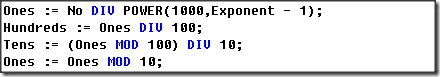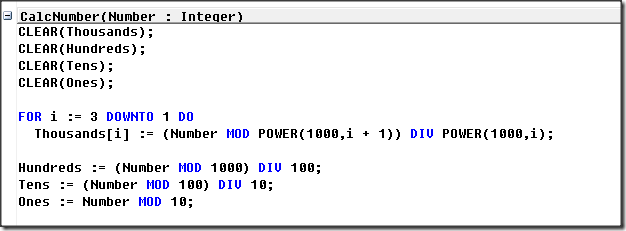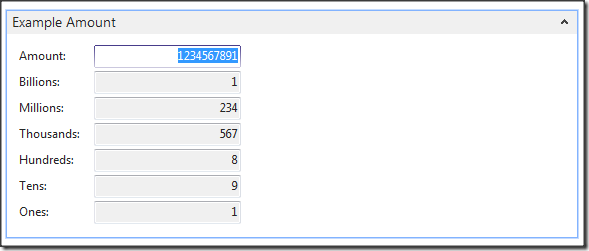# Dynamics NAV MOD and DIV Part 1

28th February 2015

In Dynamics NAV C/AL Operators are not selectable, they are used by the developers in the code, I am going to look at two of the numerical operators that are not used often MOD and DIV.

MOD and DIV are simple little functions, if you Google them you will find some convoluted answers, they are not as complicated as they might first appear, simply DIV is how many times can the number be divided Rounded to a whole number, MOD is how many units are left after a DIV, you will find a MOD function with different syntax in Excel, but you will not find the DIV function.

• DIV (Integer division) Example: 23 DIV 10 = 2;
• There are Two Tens when you Divide and Round Down the Number Twenty Three by Ten, like ROUND(23/10,1,'<‘) = 2.
• MOD (Modulus) Example: 23 MOD 10 = 3;
• When you Subtract 20 (23 DIV 10) from 23, MOD becomes the Remainder of Three, like 23 – 20 = 3.

## Where are they Used?

Report 1401 Check and Table 370 Excel Buffer come to mind, when I look at the Check Report I will see this code.### Variation

I created a Variation of the Check Code.I used a Report Request Page to display the Results of the Calculation.### Looking at the Code

POWER(Number,Multiplier) = Number Multiplied by Itself x times.

Billions := (Number MOD POWER(1000,3 + 1)) DIV POWER(1000,3);

• POWER(1000,3 + 1) = 1000000000000
• POWER(1000,3) = 1000000000
• (1234567891 MOD 1000000000000) = 1234567891
• 1234567891 DIV 1000000000 = 1

Millions := (Number MOD POWER(1000,2 + 1)) DIV POWER(1000,2);

• POWER(1000,2 + 1) = 1000000000
• POWER(1000,2) = 1000000
• (1234567891 MOD 1000000000) = 234567891
• 234567891 DIV 1000000 = 234

Thousands := (Number MOD POWER(1000,1 + 1)) DIV POWER(1000,1);

• POWER(1000,1 + 1) = 1000000
• POWER(1000,1) = 1000
• (1234567891 MOD 1000000) = 567891
• 234567891 DIV 1000 = 567

Hundreds := (Number MOD 1000) DIV 100;

• (1234567891 MOD 1000) = 891
• 234567891 DIV 100 = 8

Tens := (Number MOD 100) DIV 10;

• (1234567891 MOD 100) = 91
• 234567891 DIV 10 = 9

Ones := Number MOD 10;

• 1234567891 MOD 10 = 1

I have looked at MOD and DIV, tried to explain them in easy terms,  I have split a number into different elements, I will look in Part 2, at how I could use these Operators at item level to quantify packaging materials.

Filed in: Code Example, Tutorials